# Introduction to DiscreteFDR

Multiple testing procedures are important tools for identifying statistically significant findings in massive and complex data while controlling a specific error rate. An important focus has been given to methods controlling the false discovery rate (FDR), i.e., the expected proportion of falsely rejected hypotheses among all rejected hypotheses, which has become the standard error rate for high dimensional data analysis. Since the original procedure of Benjamini and Hochberg (1995), much effort has been undertaken to design FDR controlling procedures that adapt to various underlying structures of the data, such as the quantity of signal, the signal strength and the dependencies, among others.

The R package DiscreteFDR, presented in this paper, deals with adaptation to discrete and non-identically distributed test statics by implementing procedures developed by Döhler, Durand and Roquain (2018) (in the sequel abbreviated as [DDR]). This type of data arises in many relevant applications, in particular when data represent frequencies or counts. Examples can be found in clinical studies (see e.g., Westfall and Wolfinger (1997)), genome-wide association studies (GWAS) (see e.g., Dickhaus, Straßburger et al. (2012)) and next generation sequencing data (NGS) (see e.g., Doerge and Chen (2015)}).

# 1. A Toy example

To give a first impression of how DiscreteFDR works, we consider an artificial toy example. A more realistic example involving pharmacovigilance data is given in Section 2.

Suppose we would like to compare two treatments in nine different populations. For each population we do this by evaluating the responders and non-responders for each treatment. This leads to categorical data which can be represented, for each population $$i = 1, \ldots, 9$$ in the following 2 $$\times$$ 2 table:

Responders Non-responders
Treatment 1 $$x_{1i}$$ $$y_{1i}$$ $$n_{1i}$$
Treatment 2 $$x_{2i}$$ $$y_{2i}$$ $$n_{2i}$$
Total $$x_{1i} + x_{2i}$$ $$y_{1i} + y_{2i}$$ $$n = n_{1i} + n_{2i}$$

Denoting the responder probabilities for population $$i$$ by $$\pi_{1i}$$ and $$\pi_{2i}$$ we can test e.g.

$H_{0i}: \pi_{1i} = \pi_{2i} \qquad \text{vs.} \qquad H_{1i}: \pi_{1i} \neq \pi_{2i}$

by using Fisher’s (two-sided) exact test (see Lehmann and Romano (2006)), which is implemented in the R function fisher.test. Suppose the data in the nine populations are independent and we observe the following data frame df

library(knitr)
X1 <- c(4, 2, 2, 14, 6, 9, 4, 0, 1)
X2 <- c(0, 0, 1, 3, 2, 1, 2, 2, 2)
N1 <- rep(148, 9)
N2 <- rep(132, 9)
Y1 <- N1 - X1
Y2 <- N2 - X2
df <- data.frame(X1, Y1, X2, Y2)
kable(df, caption = "Toy Example")
Toy Example
X1 Y1 X2 Y2
4 144 0 132
2 146 0 132
2 146 1 131
14 134 3 129
6 142 2 130
9 139 1 131
4 144 2 130
0 148 2 130
1 147 2 130

In this data frame each of the 9 rows represents the data of an observed 2 $$\times$$ 2 table: e.g., the third row of the data corresponds to $$x_{13} = 2, y_{13} = 146, x_{23} = 1, y_{23} = 131$$. Even though in this example, the total number of tested hypotheses $$m = 9$$ is very small, for illustrative purposes we deal with the multiplicity problem here by controlling FDR at level $$\alpha = 5\%$$. The DBH step-down procedure can be applied directly to the data frame object df and yields an S3 object of class DiscreteFDR, for which we provide both print and summary methods:

library("DiscreteFDR")
DBH.sd.fast <- fast.Discrete(df, alternative = "two.sided", direction = "sd")
print(DBH.sd.fast)
#>
#>   Discrete Benjamini-Hochberg procedure (step-down)
#>
#> data:  df
#> number of tests = 9
#> number of rejections = 2 at global FDR level 0.05
#> (Original BH rejections = 0)
#> largest rejected p value:  0.02126871

summary(DBH.sd.fast)
#>
#>   Discrete Benjamini-Hochberg procedure (step-down)
#>
#> data:  df
#> number of tests = 9
#> number of rejections = 2 at global FDR level 0.05
#> (Original BH rejections = 0)
#> largest rejected p value:  0.02126871
#>
#>   Index    P.value   Adjusted Rejected
#> 1     4 0.01243145 0.03819796     TRUE
#> 2     6 0.02126871 0.03819796     TRUE
#> 3     1 0.12476691 0.25630985    FALSE
#> 4     8 0.22135177 0.47895996    FALSE
#> 5     5 0.28849298 0.51482782    FALSE
#> 6     2 0.49984639 1.00000000    FALSE
#> 7     9 0.60329543 1.00000000    FALSE
#> 8     7 0.68723229 1.00000000    FALSE
#> 9     3 1.00000000 1.00000000    FALSE

The output of the summary function contains the same output as the print method, but adds a table that lists the raw $$p$$-values, their adjusted counterparts and their respective rejection decision. It is sorted by raw $$p$$-values in ascending order. Our summary method actually creates a summary.DiscreteFDR object, which includes all contents of an DiscreteFDR object plus the aforementioned table. This table can be accessed directly by the $Table command. DBH.sd.fast.summary <- summary(DBH.sd.fast) DBH.sd.fast.summary$Table
#>   Index    P.value   Adjusted Rejected
#> 1     4 0.01243145 0.03819796     TRUE
#> 2     6 0.02126871 0.03819796     TRUE
#> 3     1 0.12476691 0.25630985    FALSE
#> 4     8 0.22135177 0.47895996    FALSE
#> 5     5 0.28849298 0.51482782    FALSE
#> 6     2 0.49984639 1.00000000    FALSE
#> 7     9 0.60329543 1.00000000    FALSE
#> 8     7 0.68723229 1.00000000    FALSE
#> 9     3 1.00000000 1.00000000    FALSE

Thus we can reject two hypotheses at FDR-level $$\alpha = 5\%$$. Note, that our print method also gives the number of rejections of the usual (continuous) BH procedure. In order to compare its adjusted $$p$$-values with ours, we have to determine the raw $$p$$~values first. This would be possible by applying the fisher.test function to all nine 2 $$\times$$ 2 tables. Alternatively, we may use the more convenient function fisher.pvalues.support included in our package for accessing the raw $$p$$-values. An even more simple way is to extract them from an object that contains the results:

p <- fisher.pvalues.support(df, alternative = "two.sided")
raw.pvalues <- p$raw # or: raw.pvalues2 <- DBH.sd.fast$Data$raw.pvalues all(raw.pvalues == raw.pvalues2) #>  TRUE p.adjust(raw.pvalues, method = "BH") #>  0.37430072 0.74976959 1.00000000 0.09570921 0.51928737 0.09570921 0.77313633 #>  0.49804147 0.77313633 Applying the continuous BH procedure from the stats package in the last line of code, we find that we can not reject any hypotheses at FDR-level $$\alpha = 5\%$$. Another approach of determining this is to compare the critical values of the discrete and continuous BH procedures. In the discrete case, we need the observed $$p$$-values their distributions. Both were computed by our fisher.pvalues.support function above. p <- fisher.pvalues.support(df, alternative = "two.sided") raw.pvalues <- p$raw
pCDFlist <- p$support DBH.sd.crit <- DBH(raw.pvalues, pCDFlist, 0.05, "sd", TRUE) crit.vals.BH.disc <- DBH.sd.crit$Critical.values
crit.vals.BH.cont <- 1:9 * 0.05/9
cbind(sort(raw.pvalues), crit.vals.BH.disc, crit.vals.BH.cont)
#>                  crit.vals.BH.disc crit.vals.BH.cont
#>  [1,] 0.01243145        0.01243145       0.005555556
#>  [2,] 0.02126871        0.02832448       0.011111111
#>  [3,] 0.12476691        0.03109596       0.016666667
#>  [4,] 0.22135177        0.04839433       0.022222222
#>  [5,] 0.28849298        0.05014119       0.027777778
#>  [6,] 0.49984639        0.07657062       0.033333333
#>  [7,] 0.60329543        0.07657062       0.038888889
#>  [8,] 0.68723229        0.10328523       0.044444444
#>  [9,] 1.00000000        0.10328523       0.050000000

Obviously, the critical values of the discrete approach are considerably larger than those of the continuous method. As a result, the two smallest $$p$$-values are rejected by the discrete BH procedure, because they are smaller than or equal to the respective critical values. The continuous BH approach does not reject any hypothesis, because all its critical values are smaller than the observed $$p$$-values.

For illustration, our package includes a plot method for DiscreteFDR S3 class objects. It allows to define colors, line types and point characters separately for accepted and rejected $$p$$-values and for critical values (if present in the object).

plot(DBH.sd.crit, col = c("red", "blue", "green"), pch = c(4, 2, 19), lwd = 2, type.crit = 'o',
legend = "topleft", cex = 1.3)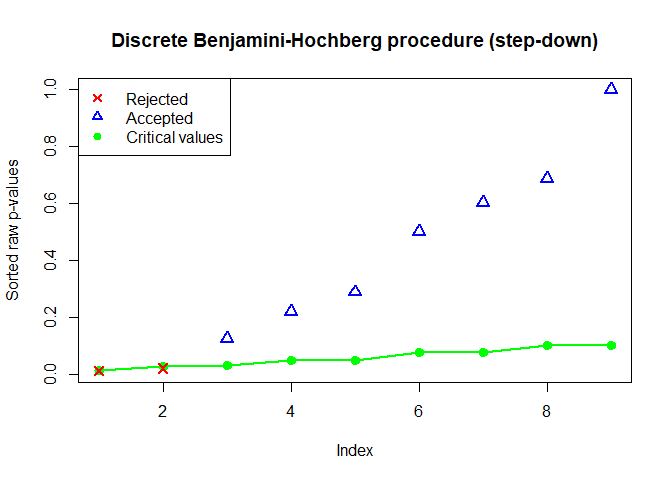Now, it is visible which observed $$p$$-values are rejected. A comparison with the continuous BH procedure could be done as follows:

plot(DBH.sd.crit, col = c("red", "blue", "green"), pch = c(4, 2, 19), lwd = 2, type.crit = 'o',
cex = 1.3, ylim = c(0, 0.25), main = "Comparison of discrete and continuous BH procedures")
points(crit.vals.BH.cont, pch = 19, cex = 1.3, lwd = 2)
legend("topright", c("Rejected", "Accepted", "Critical Values (disc.)", "Critical Values (cont.)"),
col = c("red", "blue", "green", "black"), pch = c(4, 2, 19, 19), lwd = 2, lty = 0)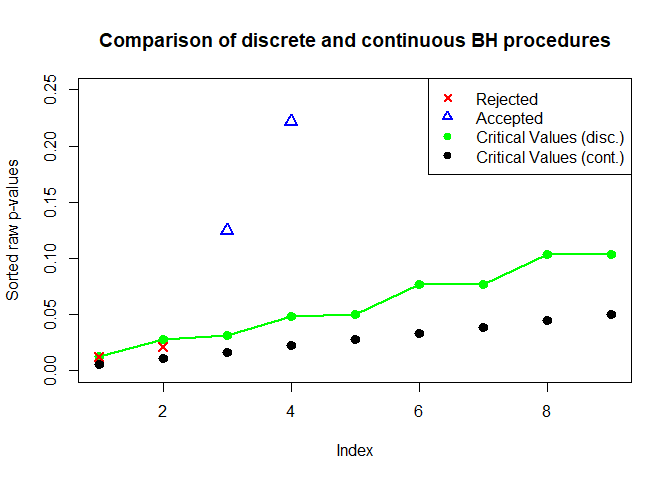As this example illustrates, the discrete approach can potentially yield a large increase in power. The gain depends on the involved distribution functions and the raw $$p$$-values. To appreciate where this comes from, it is instructive to consider the distribution functions $$F_1, \ldots, F_9$$ of the $$p$$-values under the null in more detail. Take for instance the first 2 $$\times$$ 2 table:

Responders Non-responders
Treatment 1 4 144 148
Treatment 2 0 132 132
Total 4 276 280

Fisher’s exact test works by determining the probability of observing this (or a more ‘extreme’) table, given that the margins are fixed. So each $$F_i$$ is determined by the margins of table $$i$$. Since $$x_{11} + x_{21} = 4$$, the only potentially observable tables are given by $$x_{11} = 0, \ldots, 4$$. For each one of these 5 values a $$p$$-value can be determined using the hypergeometric distribution. Therefore, the $$p$$-value of any 2 $$\times$$ 2 table with margins given by the above table can take (at most) 5 distinct values, say $$x_1, \ldots, x_5$$. Combining these 5 values into a set, we obtain the support $$\mathcal{A}_1 = \{x_1, \ldots, x_5\}$$ of distribution $$F_1$$. Now we can continue in this vein for the remaining 2 $$\times$$ 2 tables to obtain the supports $$\mathcal{A}_1, \ldots, \mathcal{A}_9$$ for the distribution functions $$F_1, \ldots, F_{9}$$. The supports can be accessed via the $support command, e.g. p$support[c(1,5)]
#> []
#>  0.04820493 0.12476691 0.34598645 0.62477763 1.00000000
#>
#> []
#>  0.002173856 0.007733719 0.028324482 0.069964309 0.154043258 0.288492981
#>  0.481808361 0.726262402 1.000000000

returns $$\mathcal{A}_1$$ and $$\mathcal{A}_5$$. Panel (a) in the following figure shows a graph of the distribution functions $$F_1, \ldots, F_9$$. Each $$F_i$$ is a step-function with $$F_i(0) = 0$$, the jumps occurring only on the support $$\mathcal{A}_i$$ and $$F_i(x) = x$$ only for $$x \in \mathcal{A}_i$$. In particular, all distributions are stochastically larger than the uniform distribution (i.e., $$F_i(x) \le x$$), but in a heterogeneous manner. This heterogeneity can be exploited e.g., by transforming the raw $$p$$-values from the exact Fisher’s test using the function $\displaystyle \xi_{\text{SD}}(x) = \sum_{i = 1}^{9} \frac{F_i(x)}{1 - F_i(x)}.$ Panel (b) shows that $$\xi_{\text{SD}}$$ is a step function. Its jumps occur on the joint support $$\mathcal{A}= \mathcal{A}_1 \cup \ldots \cup \mathcal{A}_9$$. Panel (b) also shows that $$\displaystyle \xi_{\text{SD}}(x) \ll x$$, at least for small values of $$x$$. It turns out that the critical values of our new DBH step-down procedure are essentially given by inverting $$\xi_{\text{SD}}$$ at the critical values of the [BH] procedure $$1 \cdot \alpha / 9, 2 \cdot \alpha / 9, \ldots, \alpha$$, so that these values are considerably larger than the [BH] critical values. This is illustrated in panel (c) along with the ordered $$p$$~values. In particular, all asterisks are located above the green [BH] dots, therefore this procedure can not reject any hypothesis. In contrast, the two smallest $$p$$~values are located below red DBH step-down dots, so that this procedure rejects two hypotheses as we had already seen earlier.

pCDFlist <- p$support stepf <- lapply(pCDFlist, function(x) stepfun(x, c(0, x))) par(mfcol = c(1, 3), mai = c(1, 0.5, 0.3, 0.1)) plot(stepf[], xlim = c(0, 1), ylim = c(0, 1), do.points = FALSE, lwd = 1, lty = 1, ylab = "F(x)", main = "(a)") for(i in (2:9)){ plot(stepf[[i]], add = TRUE, do.points = FALSE, lwd = 1, col = i) } segments(0, 0, 1, 1, col = "grey", lty = 2) # Plot xi support <- sort(unique(unlist(pCDFlist))) components <- lapply(stepf, function(s){s(support) / (1 - s(support))}) xi.values <- 1/9 * Reduce('+', components) xi <- stepfun(support, c(0, xi.values)) plot(xi, xlim = c(0, 0.10), ylim = c(0, 0.10), do.points = FALSE, ylab = expression(xi), main = "(b)") segments(0, 0, 0.1, 0.1, col = "grey", lty = 2) # Plot discrete critical values as well a BH constants and raw p-values DBH.sd <- DBH(raw.pvalues, pCDFlist, direction = "sd", ret.crit.consts = TRUE) plot(DBH.sd, col = c("black", "black", "red"), pch = c(4, 4, 19), type.crit = 'p', ylim = c(0, 0.15), cex = 1.3, main = "(c)", ylab = "Critical Values") points(1:9, 0.05 * (1:9) / 9, col = "green", pch = 19, cex = 1.3) mtext("Figure 1", 1, outer = TRUE, line = -2)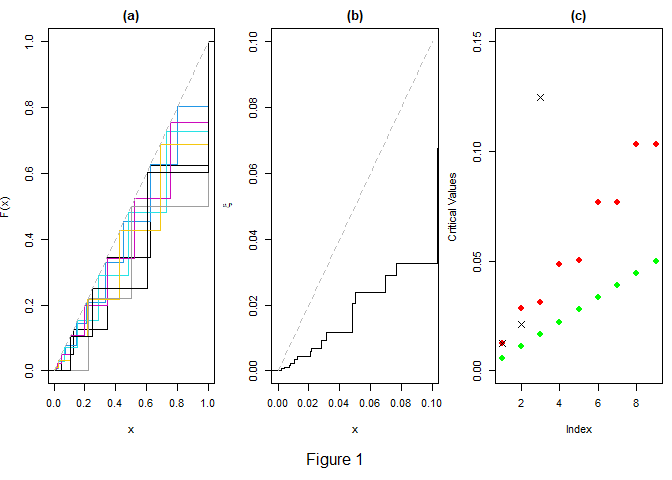Panel (a) depicts the distribution functions $$F_1, \ldots, F_9$$ in various colors, (b) is a graph of the transformation $$\xi_{\text{SD}}$$. The uniform distribution function is shown in light grey in (a) and (b). Panel (c) shows the [BH] critical values (green dots), the DBH step-down critical values (red dots) and the sorted raw $$p$$-values (asterisks). # 2. Further Analyses ## 2.1. Analysis of Pharmacovigilance Data To illustrate how the procedures in DiscreteFDR can be used for real data, we revisit the analysis of the pharmacovigilance data from Heller and Gur (2011) performed in [DDR]. This data set is obtained from a database for reporting, investigating and monitoring adverse drug reactions due to the Medicines and Healthcare products Regulatory Agency in the United Kingdom. It contains the number of reported cases of amnesia as well as the total number of adverse events reported for each of the $$m = 2446$$ drugs in the database. For more details we refer to Heller and Gur (2011) and to the accompanying R-package discreteMTP (Heller et al. (2012)), which also contains the data. Heller and Gur (2011) investigate the association between reports of amnesia and suspected drugs by performing for each drug a Fisher’s exact test (one-sided) for testing association between the drug and amnesia while adjusting for multiplicity by using several (discrete) FDR procedures. In what follows we present code that reproduces parts of Figure 2 and Table 1 in [DDR]. We proceed as in the example in Section 1. Since we need to access the critical values, we first determine the $$p$$-values and their support for the data set amnesia contained for convenience in the package DiscreteFDR. For this, we use the option "HG2011" in the function fisher.pvalues.support. data(amnesia) amnesia.formatted <- fisher.pvalues.support(amnesia[, 2:3], input = "HG2011") raw.pvalues <- amnesia.formatted$raw
pCDFlist <- amnesia.formatted$support Then we perform the FDR analysis with functions DBH and ADBH (SU and SD) and DBR at level $$\alpha = 0.05$$ including critical values. DBH.su <- DBH(raw.pvalues, pCDFlist, ret.crit.consts = TRUE) DBH.sd <- DBH(raw.pvalues, pCDFlist, direction = "sd", ret.crit.consts = TRUE) ADBH.su <- ADBH(raw.pvalues, pCDFlist, ret.crit.consts = TRUE) ADBH.sd <- ADBH(raw.pvalues, pCDFlist, direction = "sd", ret.crit.consts = TRUE) DBR.su <- DBR(raw.pvalues, pCDFlist, ret.crit.consts = TRUE) It is helpful to have a histogram of the observed $$p$$-pvalues. hist(DBH.sd)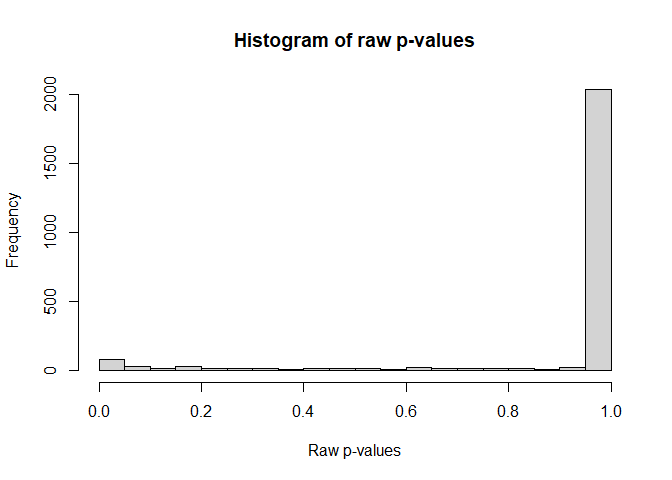This histogram indicates a highly discrete $$p$$~value distribution, which strongly suggests the use of discrete methods. By accessing the critical values we can now generate a plot similar to Figure 2 from [DDR]. Note that both [DBH-SU] and [DBH-SD] are visually indistinguishable from their adaptive counterparts. m <- length(raw.pvalues) crit.values.BH <- 0.05 * (1:m) / m scale.points <- 0.7 plot(DBH.su, col = c("black", "black", "orange"), pch = NA, type.crit = 'p', xlim = c(1, 100), ylim = c(0, DBH.su$Critical.values), ylab = "critical values", cex = scale.points, main = "")

points(crit.values.BH[1:105],          col = "green",  pch = 19, cex = scale.points)
points(DBH.sd$Critical.values[1:105], col = "red", pch = 19, cex = scale.points) points(ADBH.su$Critical.values[1:105], col = "blue",   pch = 19, cex = scale.points)
points(ADBH.sd$Critical.values[1:105], col = "purple", pch = 19, cex = scale.points) points(DBR.su$Critical.values[1:105],  col = "yellow", pch = 19, cex = scale.points)
points(sort(raw.pvalues),                              pch = 4,  cex = scale.points)
mtext("Figure 2", 1, outer = TRUE, line = -1)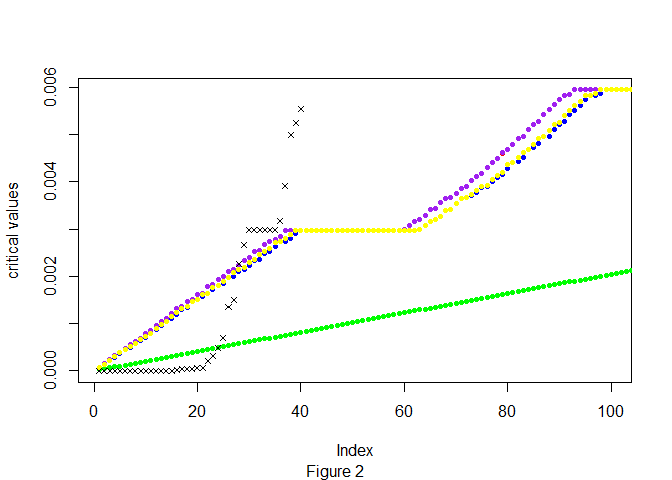Critical values for [BH] (green dots), [DBH-SU] (orange dots), [DBH-SD] (red dots), [A-DBH-SU] (blue dots), [A-DBH-SD] (purple dots), [DBR] (yellow dots). The sorted raw $$p$$-values are represented by asterisks.

The rejected hypotheses can be accessed via the command $Indices. The following code yields some of the values from Table 1 in [DDR]: rej.BH <- length(which(p.adjust(raw.pvalues, method = "BH") <= 0.05)) rej.DBH.su <- length(DBH.su$Indices)
rej.DBH.sd <- length(DBH.sd$Indices) rej.ADBH.su <- length(ADBH.su$Indices)
rej.ADBH.sd <- length(ADBH.sd$Indices) rej.DBR.su <- length(DBR.su$Indices)
c(rej.BH, rej.DBH.su, rej.DBH.sd, rej.ADBH.su, rej.ADBH.sd, rej.DBR.su)
#>  24 27 27 27 27 27

The (continuous) BH rejects only 24 hypotheses whereas all the discrete procedures implemented in DiscreteFDR are able to identify three additional drug candidates potentially associated with amnesia.

## 2.2. Other Types of Discrete Tests

In this section we sketch how can be used to analyze arbitrary multiple discrete tests. Jiménez-Otero et al. (2018) used DiscreteFDR to detect disorder in NGS experiments based on one-sample tests of the Poisson mean. Rather than reproducing their analysis in detail, we illustrate the general approach by using a toy example similar to the one in Section 1 and show how the test of the Poisson mean can be accommodated by DiscreteFDR.

To fix ideas, suppose we observe $$m = 9$$ independent Poisson distributed counts $$N_1, \ldots, N_9$$ (Jiménez-Otero et al. (2018) used this to model the read counts of different DNA bases). We assume that $$N_i \sim \text{Pois}(\lambda_i)$$ and the goal is to identify cases where $$\lambda_i$$ is larger than some pre-specified value $$\lambda^0_i$$, i.e., we have the (one-sided) multiple testing problem $H_{0i}: \lambda_i = \lambda^0_i \qquad \text{vs.} \qquad H_{1i}: \lambda_i > \lambda^0_i.$ As in Section 1, the goal is to adjust for multiple testing by using the [DBH-SD] procedure at FDR-level $$\alpha = 5\%$$. In our example the observations $$n_1,\ldots, n_9$$ and parameters $$\lambda^0_1, \ldots, \lambda^0_9$$ are given as follows:

lambda.0 <- c(0.6, 1.2, 0.7, 1.3, 1.0, 0.2, 0.8, 1.3, 0.9)
lambda.vector <- lambda.0
observations <- c(3, 3, 1, 2, 3, 3, 1, 2, 4)
configuration <- cbind(observations, lambda.0)
alpha <- 0.05
m <- length(observations)
print(configuration)
#>       observations lambda.0
#>  [1,]            3      0.6
#>  [2,]            3      1.2
#>  [3,]            1      0.7
#>  [4,]            2      1.3
#>  [5,]            3      1.0
#>  [6,]            3      0.2
#>  [7,]            1      0.8
#>  [8,]            2      1.3
#>  [9,]            4      0.9

Denote by $$G_i$$ the distribution of $$N_i$$ under $$H_{0i}$$ i.e., $$G_i(x) = P(N_i \le x)$$. For observations $$n_1,\ldots, n_9$$ of $$N_1, \ldots, N_9$$ the $$p$$-values for the above one-sided test are given by $p_i = P(N_i \ge n_i) = P(N_i > n_i - 1) = \overline{G_i}(n_i - 1),$ where $$\overline{G_i}(x) = P(N_i > x) = 1 - G_i(x)$$ denotes the survival function of the Poisson distribution with parameter $$\lambda^0_i$$. Thus the raw $$p$$-values are determined by the following R code:

raw.pvalues <- sapply(1:m,function(i){ppois(observations[i] - 1, lambda.vector[i], lower.tail = FALSE)})
print(raw.pvalues)
#>  0.023115288 0.120512901 0.503414696 0.373176876 0.080301397 0.001148481
#>  0.550671036 0.373176876 0.013458721

Following the definition of the function in R we define the inverse function of $$\overline{G_i}$$ by $\left(\overline{G_i}\right)^{-1}(p) = \min\{n \in \mathbb{N}: \overline{G_i}(n) \le p\}$ and obtain for the distribution function of the $$i$$-th $$p$$-value under the null $F_i(x) = \overline{G_i}\left(\left(\overline{G_i}\right)^{-1}(x)\right).$ Each function $$F_i$$ is a step function with $$F_i(0) = 0$$, $$F_i(1) = 1$$ and there exists an infinite sequence of jumps at locations $$1 = x_1 > x_2 > \ldots > x_n > x_{n + 1} > \ldots > 0$$ such that $$F(x_j) = x_j$$ for $$j \in \mathbb{N}$$.

Initially it seems that we run into a problem if we want to determine the critical values of [DBH-SD] since the supports of $$F_1, \ldots, F_9$$ are no longer finite (but still discrete). We can deal with this problem by using the observation from Section that it is sufficient to consider new, restricted supports $$\mathcal{A}_i \cap [s^{\tiny \mbox{min}},1]$$ where the lower threshold satisfies \begin{align} s^{\tiny \mbox{min}} &\le \tau^{\tiny \mbox{min}}_1 = \max \left\{t \in \mathcal{A}\::\: t \leq y^{\tiny \mbox{min}} \right\} \qquad \text{where} \qquad y^{\tiny \mbox{min}} = \frac{\alpha}{m} \cdot \left(1 + \frac{\alpha}{m} \right)^{-1}. \end{align} To determine such an $$s^{\tiny \mbox{min}}$$ we proceed as follows. Define $$n^{\tiny \mbox{max}}_i = \left(\overline{G_i}\right)^{-1}(y^{\tiny \mbox{min}}) + 1$$, $$t^{\tiny \mbox{min}}_i = \overline{G_i}(n^{\tiny \mbox{max}}_i - 1)$$ and set $$s^{\tiny \mbox{min}} = \min\left(t^{\tiny \mbox{min}}_1, \ldots, t^{\tiny \mbox{min}}_9 \right)$$. It is easily checked that this choice of $$s^{\tiny \mbox{min}}$$ satisfies the above equation. We can determine $$s^{\tiny \mbox{min}}$$ by the following code

y.min <- alpha/m * (1 + alpha/m)^(-1)
n.max <- sapply(1:m, function(w){qpois(y.min,        lambda.vector[w], lower.tail = FALSE)}) + 1
t.min <- sapply(1:m, function(w){ppois(n.max[w] - 1, lambda.vector[w], lower.tail = FALSE)})
s.min <- min(t.min)
print(s.min)
#>  0.0007855354

For determining the restricted supports it is actually more convenient to work with $$n^{\tiny \mbox{max}}_i$$ than $$s^{\tiny \mbox{min}}$$. We can subsequently use these supports as the pCDFlist argument in the usual way when calling the DBH function:

supports <- lapply(1:m, function(w){sort(ppois(0:n.max[w] - 1, lambda.vector[w], lower.tail = FALSE))})
DBH.sd <- DBH(raw.pvalues, supports, direction = "sd", ret.crit.consts = TRUE)

Figure 3 shows a summary similar to Figure 1. Applying the continuous BH procedure

p.adjust(raw.pvalues, method = "BH")
#>  0.06934586 0.21692322 0.55067104 0.47979884 0.18067814 0.01033633 0.55067104
#>  0.47979884 0.06056424

results in one rejection at FDR-level $$\alpha = 5\%$$, whereas the DBH step-up procedure can reject three hypotheses:

DBH.sd\$Adjusted
#>  0.039602625 0.101622881 0.580898946 0.522450788 0.101509307 0.001935955
#>  0.626257875 0.522450788 0.033073393

This information can also be obtained by our print method:

print(DBH.sd)
#>
#>   Discrete Benjamini-Hochberg procedure (step-down)
#>
#> data:  raw.pvalues and pCDFlist
#> number of tests = 9
#> number of rejections = 3 at global FDR level 0.05
#> (Original BH rejections = 1)
#> largest rejected p value:  0.02311529

As in Figure 1, Panel (c) presents a graphical comparison between the two procedures applied to the $$p$$-values.

stepf <- lapply(supports, function(x) stepfun(x, c(0, x)))
par(mfcol = c(1, 3), mai = c(1, 0.5, 0.3, 0.1))

plot(stepf[], xlim = c(0,1), ylim = c(0,1), do.points = FALSE, lwd = 1, lty = 1, ylab = "F(x)",
main = "(a)")
for(i in (2:9)){
plot(stepf[[i]], add = TRUE, do.points = FALSE, lwd = 1, col = i)
}
segments(0, 0, 1, 1, col = "grey", lty = 2)

#   Plot xi
support <- sort(unique(unlist(supports)))
components <- lapply(stepf, function(s){s(support) / (1 - s(support))})
xi.values <- 1/9 * Reduce('+', components)
xi <- stepfun(support, c(0, xi.values))
plot(xi, xlim = c(0, 0.10), ylim = c(0, 0.10), do.points = FALSE, ylab = expression(xi), main = "(b)")
segments(0, 0, 0.1, 0.1, col = "grey", lty = 2)

#   Plot discrete critical values as well a BH constants
DBH.sd <- DBH(raw.pvalues, supports, direction = "sd", ret.crit.consts = TRUE)
plot(DBH.sd, col = c("black", "black", "red"), pch = c(4, 4, 19), type.crit = 'p', ylim = c(0, 0.15),
cex = 1.3, main = "(c)", ylab = "Critical Values")
points(1:9, 0.05 * (1:9) / 9, col = "green", pch = 19, cex = 1.3)

mtext("Figure 3", 1, outer = TRUE, line = -2)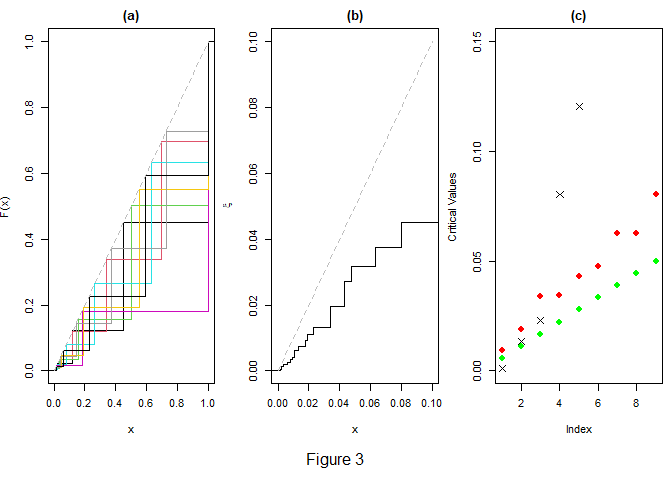Panel (a) depicts the distribution functions $$F_1, \ldots, F_9$$ in various colors, (b) is a graph of the transformation function $$\xi_{\text{SD}}$$. The uniform distribution function is shown in light grey in (a) and (b). Panel (c) shows the [BH] critical values (green dots), the DBH step-down critical values (red dots) and the sorted raw $$p$$-values (asterisks).